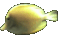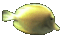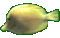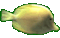Graphs of Circles in Center Radius Form     Math Help     Game Tips:

- The circle represented by x2+y2=r2 has the center point P(0,0) and has the radius r units.

- The circle represented by (x-h)2+(y-k)2=r2 has the center point P(h,k) and has the radius r units.

- When comparing the circles x2+y2=32 and (x-4)2+(y-1)2=32, the two circles have the same size radius=3
and (x-4)2+(y-1)2=32 is shifted four units East and one unit North compared to x2+y2=32.
The circle (x-4)2+(y-1)2=32 has the center (4,1).

- When comparing the circles x2+y2=9 and (x+5)2+(y-1)2=9, the two circles have the same size radius=3
and (x+5)2+(y-1)2=9 is translated five units in the negative x direction
and translated one unit in the positive y direction compared to x2+y2=9.
The circle (x+5)2+(y-1)2=9 has the center (-5,1) and the radius r=3.

- Your Game Score is reduced by the number of fish hits.

- To slow the game speed repeat tap/click on the word Slider.
- To increase the game speed repeat tap/click on the word Math.
- Speed can also be adjusted with a keyboard's - and + keys.

- Refresh/Reload the web page to restart the game.